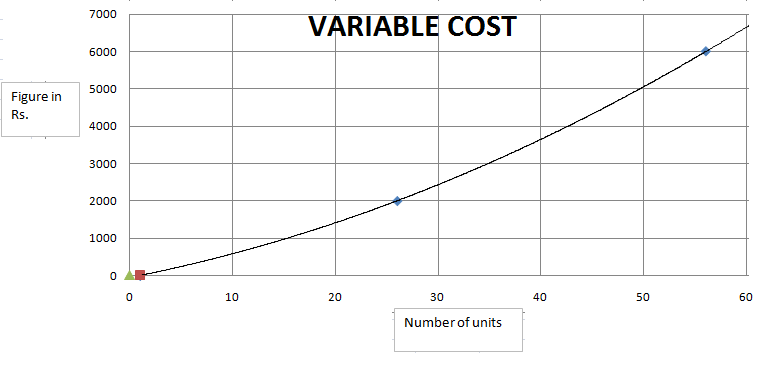### CAT 2000 Question Paper Question 34

Instructions

Answer these questions based on the following Information:

ABC Ltd. produces widgets for which the demand is unlimited and they can sell all of their production. The graph below describes the monthly variable costs incurred by the company as a function of the quantity produced. In addition, operating the plant for one shift results in a fixed monthly cost of Rs. 800. Fixed monthly costs for second shift operation are estimated at Rs. 1200. Each shift operation provides capacity for producing 30 widgets per month.
Note: Average unit cost, AC = Total monthly costs/monthly production, and Marginal cost MC is the rate of change in total cost for unit change in quantity produced.Question 34

# From the data provided it can be inferred that, for production levels in the range of 0 to 60 units,

Solution

As the graph is an increasing function, MC will always increase for increase in number of units.

• All Quant CAT Formulas and shortcuts PDF
• 30+ CAT previous papers with solutions PDF